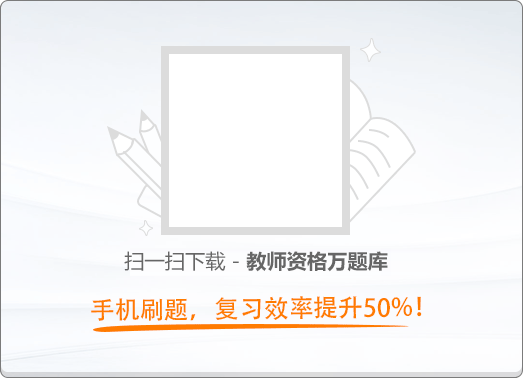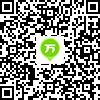120:002019年3月《幼儿综合素质》真题

1
(单项选择题)

• A.

阶段性

• B.

整体性

• C.

个体差异性

• D.

独特性

• A
• B
• C
• D

2
(单项选择题)

• A.

正确，有利于凸显幼儿园特色

• B.

不正确，不利于幼儿知识学习

• C.

正确，有利于培养幼儿艺术特长

• D.

不正确，不利于促进幼儿全面发展

• A
• B
• C
• D

3
(单项选择题)

• A.

错误，忽视了幼儿动作发展

• B.

错误，不能讽刺挖苦幼儿

• C.

正确，提高了幼儿绘画能力

• D.

正确，应该严格要求幼儿

• A
• B
• C
• D

4
(单项选择题)

• A.

“辰辰，不准玩椅子！”

• B.

“辰辰，你有多动症吗”

• C.

“辰辰请坐好，椅子会坏的！”

• D.

“辰辰请坐好，你会摔跤的”

• A
• B
• C
• D

5
(单项选择题)

• A.

集体所有制经济

• B.

非公有制经济

• C.

互联网经济

• D.

国有经济

• A
• B
• C
• D

6
(单项选择题)

• A.

受教育权

• B.

人格尊严权

• C.

人身自由权

• D.

生命健康权

• A
• B
• C
• D

7
(单项选择题)

• A.

国家公务员制度

• B.

教育应员制度

• C.

教育职员制度

• D.

教育公务员制度

• A
• B
• C
• D

8
(单项选择题)

• A.

同级人民政府或上一级人民政府有关部门

• B.

所在地区中级人民法院或省高级人民法院

• C.

所在地区人民检察院或最高人民检察院

• D.

上一级人民政府或中央人民政府有关部门

• A
• B
• C
• D

9
(单项选择题)

• A.

予以关闭，依法吊销营业执照

• B.

责令改正，依法给予行政处罚

• C.

予以查封，依法没收违法所得

• D.

责令停业，依法追究民事责任

• A
• B
• C
• D

10
(单项选择题)

• A.

对玲玲的家长进行批评教育

• B.

向当地公安机关报案

• C.

对玲玲的家长处以一定罚款

• D.

向当地法院提起诉讼

• A
• B
• C
• D

11
(单项选择题)

• A.

合法，教师可以喂食非处方药

• B.

合法，有利于防止疾病传播扩散

• C.

不合法，幼儿用药应先征得监护人同意

• D.

不合法，幼儿园应在医师的指导下用药

• A
• B
• C
• D

12
(单项选择题)

• A.

给予批评教育，督促期限改正

• B.

给予行政处分，责令赔礼道歉

• C.

做好协助工作，督促家长送雯雯接受义务教育

• D.

采取强制措施，责令家长送雯雯接受义务教育

• A
• B
• C
• D

13
(单项选择题)

• A.

接受意见，适当增加拼音和写字的内容

• B.

听取意见，耐心向家长分析不教的原因

• C.

尊重家长，推荐校外辅导机构

• D.

不予理会，尊重家长的转园自由

• A
• B
• C
• D

14
(单项选择题)

• A.

不正确，没有维护幼儿的同伴关系

• B.

不正确，没有做到对幼儿一视同仁

• C.

正确，有利于良好家园关系的建立

• D.

正确，有利于获得更多的办园资源

• A
• B
• C
• D

15
(单项选择题)

• A.

引导幼儿逐渐学会自我约束

• B.

对吵闹的幼儿进行说服教育

• C.

让家长接吵闹孩子回家安抚

• D.

引导幼儿参与感兴趣的活动

• A
• B
• C
• D

16
(单项选择题)

• A.

有利于保护幼儿的自尊心

• B.

有利于提高幼儿的操作能力

• C.

有利于增强幼儿的秩序感

• D.

有利于培养幼儿的时间观念

• A
• B
• C
• D

17
(单项选择题)• A.

阿波罗

• B.

波塞冬

• C.

阿瑞斯

• D.

雅典娜

• A
• B
• C
• D

18
(单项选择题)

• A.

金星轨道和地球轨道之间

• B.

地球轨道和火星轨道之间

• C.

火星轨道和木星轨道之间

• D.

木星轨道和土星轨道之间

• A
• B
• C
• D

19
(单项选择题)

• A.

拉瓦锡

• B.

玻意耳

• C.

普利斯特利

• D.

阿伏伽德罗

• A
• B
• C
• D

20
(单项选择题)

• A.

开普勒

• B.

哥白尼

• C.

第谷

• D.

牛顿

• A
• B
• C
• D

21
(单项选择题)

• A.

《闪闪的红星》

• B.

《渡江使察记》

• C.

《南征北战》

• D.

《英雄儿女》

• A
• B
• C
• D

22
(单项选择题)

• A.

舒曼

• B.

贝多芳

• C.

勃拉姆斯

• D.

柴科夫斯基

• A
• B
• C
• D

23
(单项选择题)

• A.

刘邦　项羽

• B.

曹操　袁绍

• C.

蒋坚　谢安

• D.

孙斌　庞涓

• A
• B
• C
• D

24
(单项选择题)

• A.

《桃花扇》

• B.

《南柯梦》

• C.

《牡丹亭》

• D.

《长生殿》

• A
• B
• C
• D

25
(单项选择题)• A.

葫芦娃（《葫芦兄弟》）

• B.

阿童木（《铁壁阿童木》）

• C.

一休（《聪明的一休》）

• D.

哪吒（《哪吒闹海》）

• A
• B
• C
• D

26
(单项选择题)

• A.

通过多文档窗口操作，文档窗口可以拆分成为两个文档窗口

• B.

多个文档编键工作结束，只能全部存盘后才可关闭文档窗口

• C.

允许同时打开多个文档进行编辑，每个文档有一个文档窗口

• D.

多个文档窗口的内容之间可以进行剪切、粘贴和复制等操作

• A
• B
• C
• D

27
(单项选择题)

• A.

可以动态显示文本和对象

• B.

可以设置幻灯片切换效果

• C.

图表不可以设置动画效果

• D.

可以更改动画对象的出现顺序

• A
• B
• C
• D

28
(单项选择题)

• A.

飞机票—船票

• B.

戏票—入场券

• C.

购水票—门票

• D.

餐券—优惠券

• A
• B
• C
• D

29
(单项选择题)

• A.

122

• B.

124

• C.

126

• D.

128

• A
• B
• C
• D